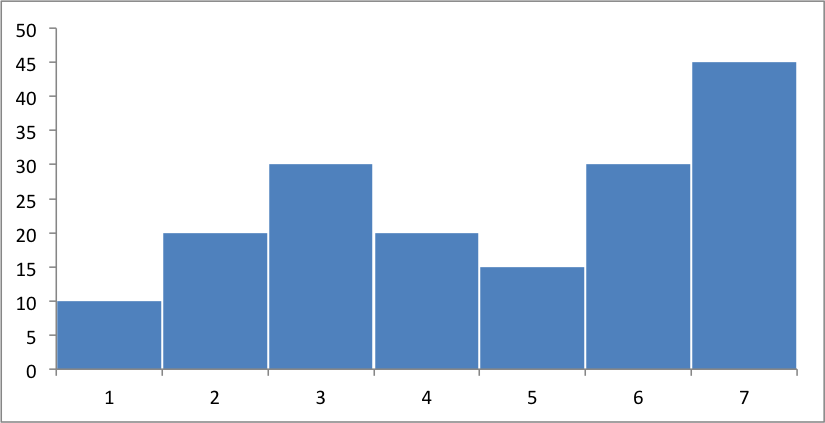# Example: Column Chart

This program is an example of creating a simple column chart:```##############################################################################
#
# An example of creating a chart with Pandas and XlsxWriter.
#
# Copyright 2013, John McNamara, jmcnamara@cpan.org
#

import pandas as pd

# Some sample data to plot.
list_data = [10, 20, 30, 20, 15, 30, 45]

# Create a Pandas dataframe from the data.
df = pd.DataFrame(list_data)

# Create a Pandas Excel writer using XlsxWriter as the engine.
excel_file = 'column.xlsx'
sheet_name = 'Sheet1'

writer = pd.ExcelWriter(excel_file, engine='xlsxwriter')
df.to_excel(writer, sheet_name=sheet_name)

# Access the XlsxWriter workbook and worksheet objects from the dataframe.
# This is equivalent to the following using XlsxWriter on its own:
#
#    workbook = xlsxwriter.Workbook('filename.xlsx')
workbook = writer.book
worksheet = writer.sheets[sheet_name]

# Create a chart object.

# Configure the series of the chart from the dataframe data.
'values':     '=Sheet1!\$B\$2:\$B\$8',
'gap':        2,
})

# You can also use array notation to define the chart values.
#        'values':     ['Sheet1', 1, 1, 7, 1],
#        'gap':        2,
#    })

# Configure the chart axes.
chart.set_y_axis({'major_gridlines': {'visible': False}})

# Turn off chart legend. It is on by default in Excel.
chart.set_legend({'position': 'none'})

# Insert the chart into the worksheet.
worksheet.insert_chart('D2', chart)

# Close the Pandas Excel writer and output the Excel file.
writer.save()
```